## What is Binary Decoders? | How it Works?# What is Binary Decoders? | How it Works?

When we design large storage devices we need ways too quickly and easily retrieve information from specific locations in the storage devices. We create this retrieval system by storing information in chunks of bits that are stored at addresses and we encode the details of these addresses are within a binary code. So, we need a circuit that decodes these binary codes and activates one memory address at a time this circuit is called a binary decoder.

Imagine with me that we need to manipulate a memory device that has eight memory addresses. To uniquely encode each address we need three address bits.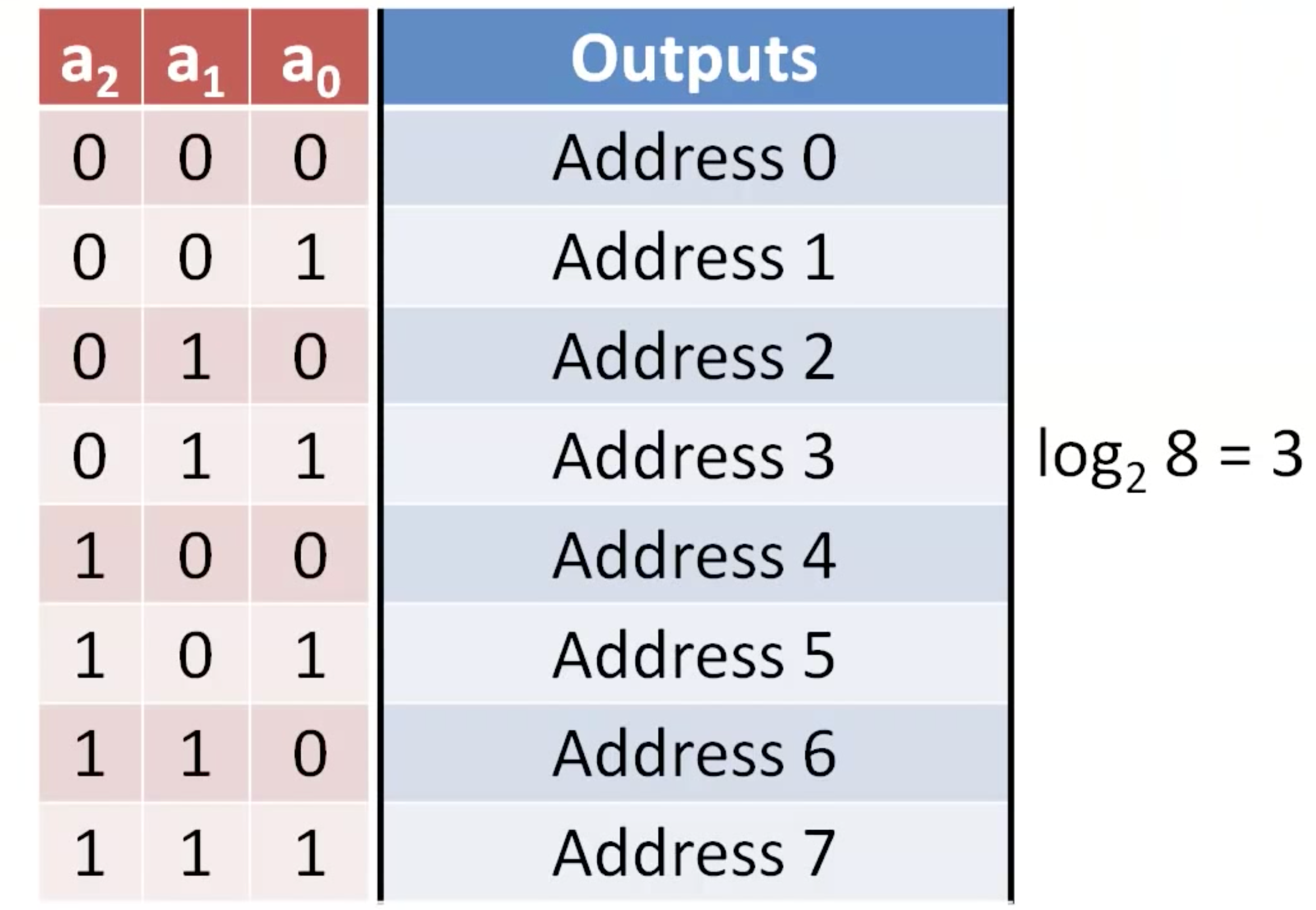To make the addressing simple we use unsigned binary numbers as our code for the addresses. Now what we want to create is a decoder circuit that will send a high voltage out on one line at a time, So that exactly one address is activated at a time.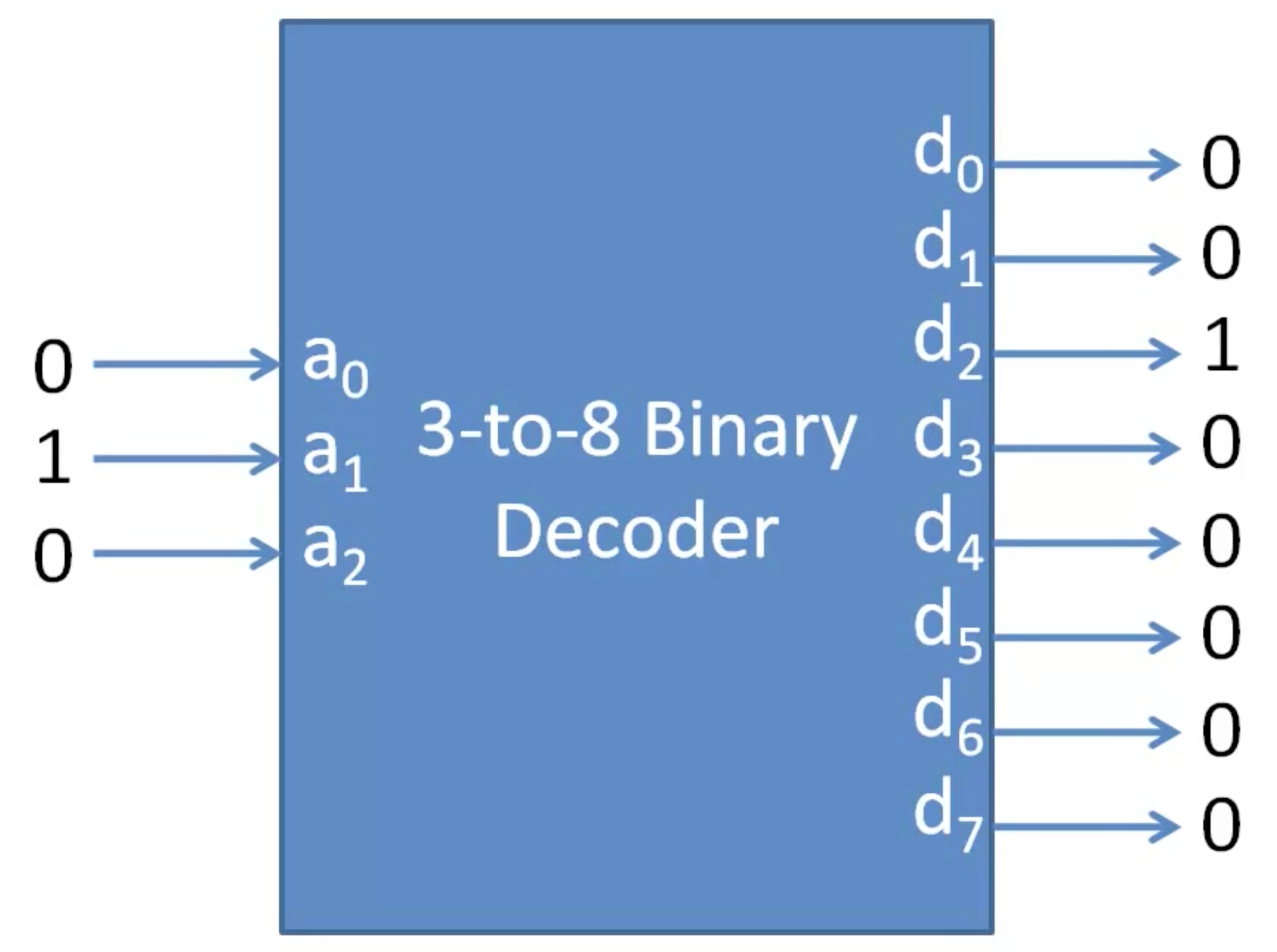So our binary decoder will have N inputs and 2(N) outputs. To create each of these outputs we will need to implement every minterm. So, we will need every variable and it’s complement.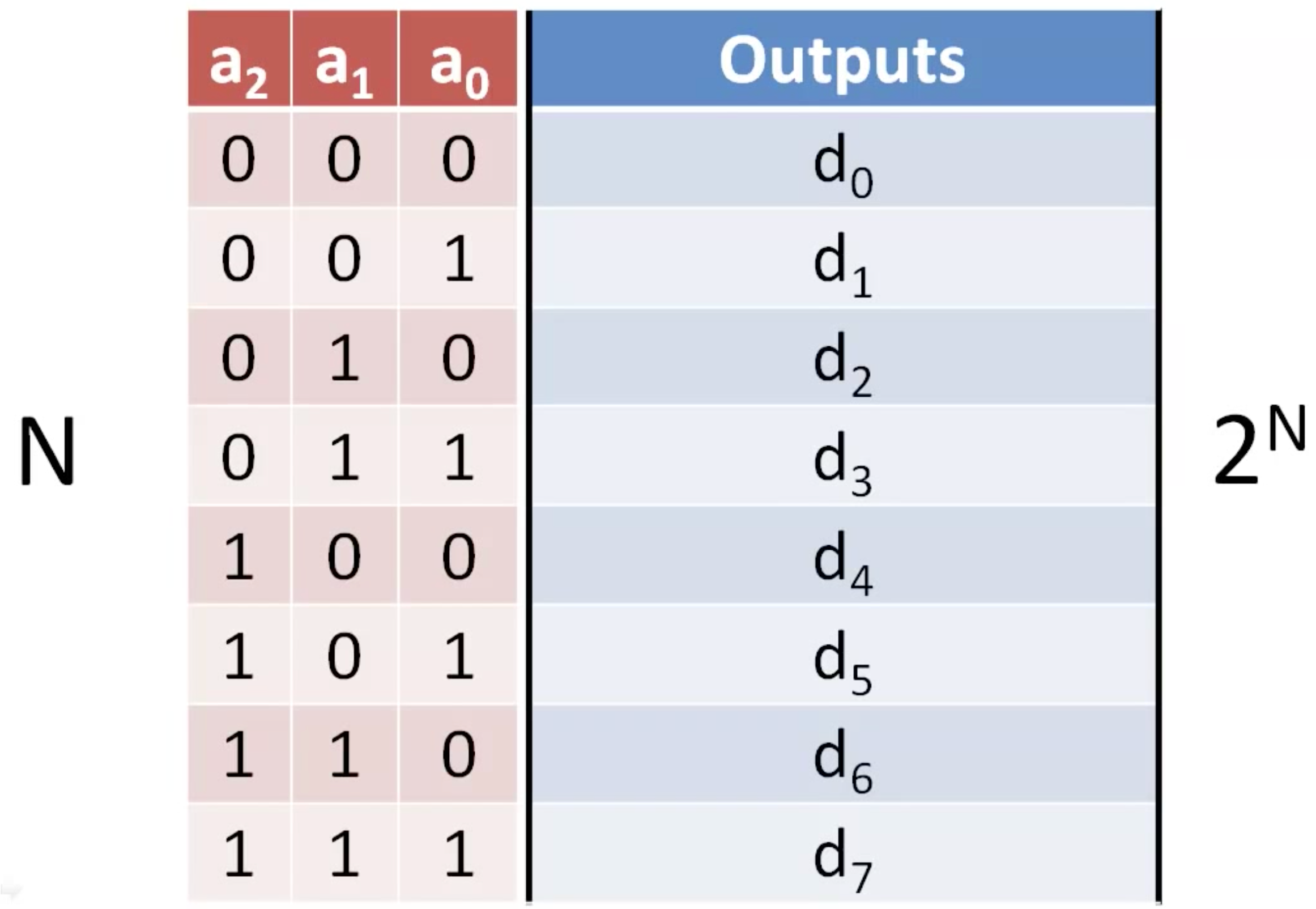Then will AND these literals together to create each minterm. For example, for minterm zeros will implement ever complemented variable into the AND gate will similarly design every other minterm. We call this circuit a binary decoder.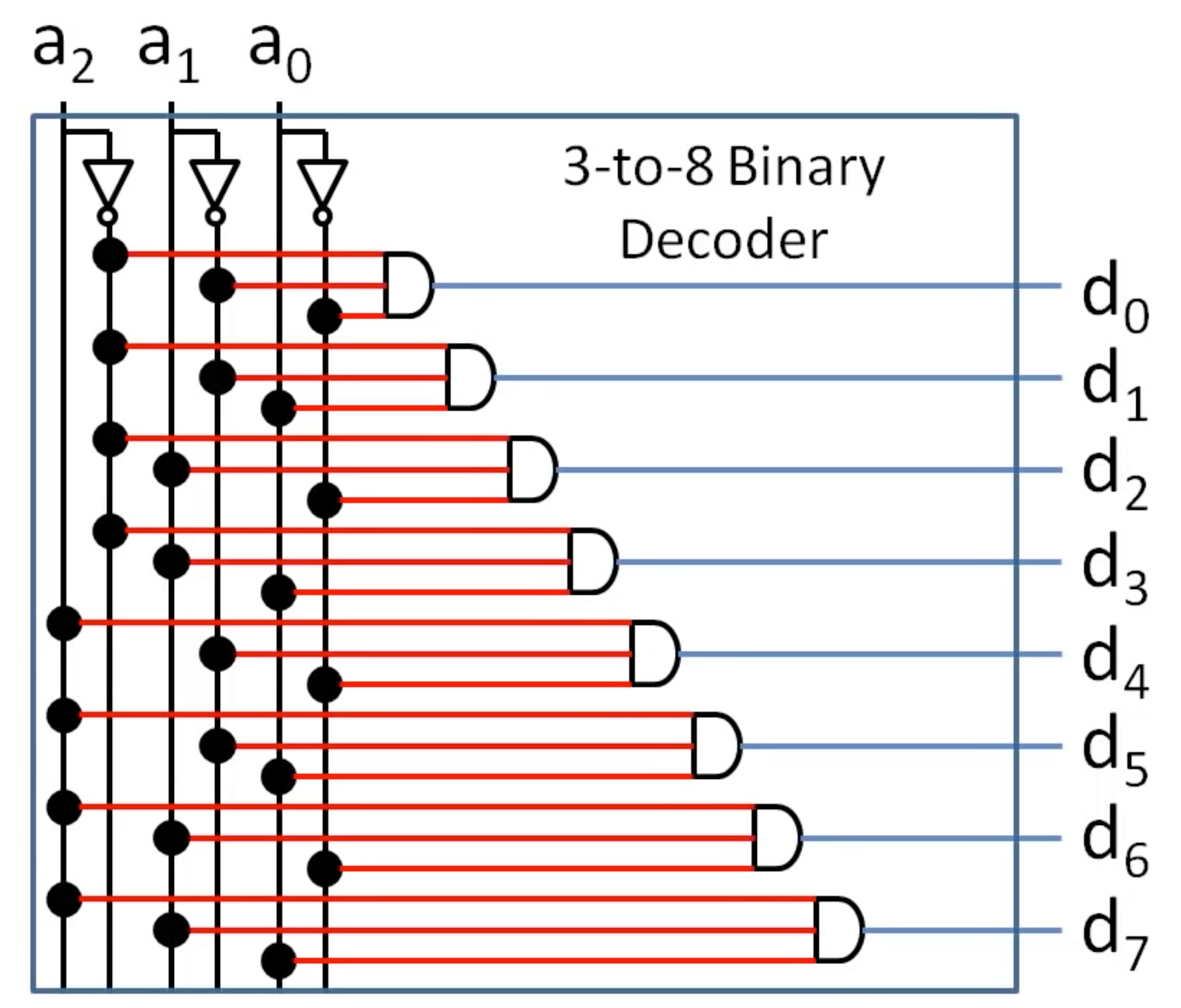Because it decodes a binary input into a series of ones and zeros on a set of data outputs. The first number in the name tells us about the number of bits in the binary code, And a second number in the name tells us about the number of data lines that are decoded. Because each data line corresponds to a different minterm. Only one of the data lines will be one at any time.Once we have designed a binary decoder we abstract its content and represent it with a box that receives a binary code and outputs a set of data outputs that correspond to the different midterms.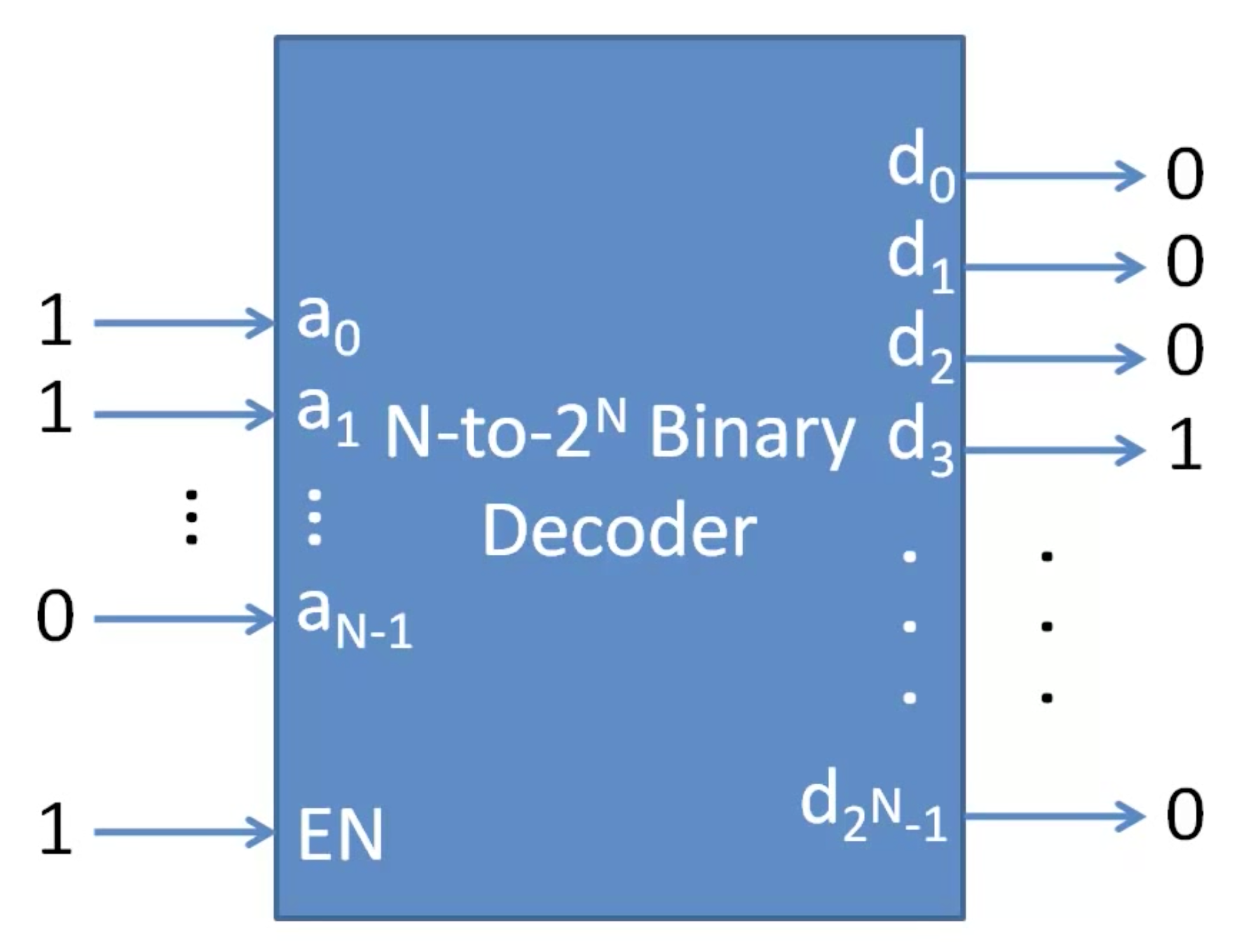Generally, each binary decoder will have an input and to the end outputs. In some applications, we also add an enable input bit to the decoder when the enable bit is 1, When the enable bit is 0 all of the decoder output 0.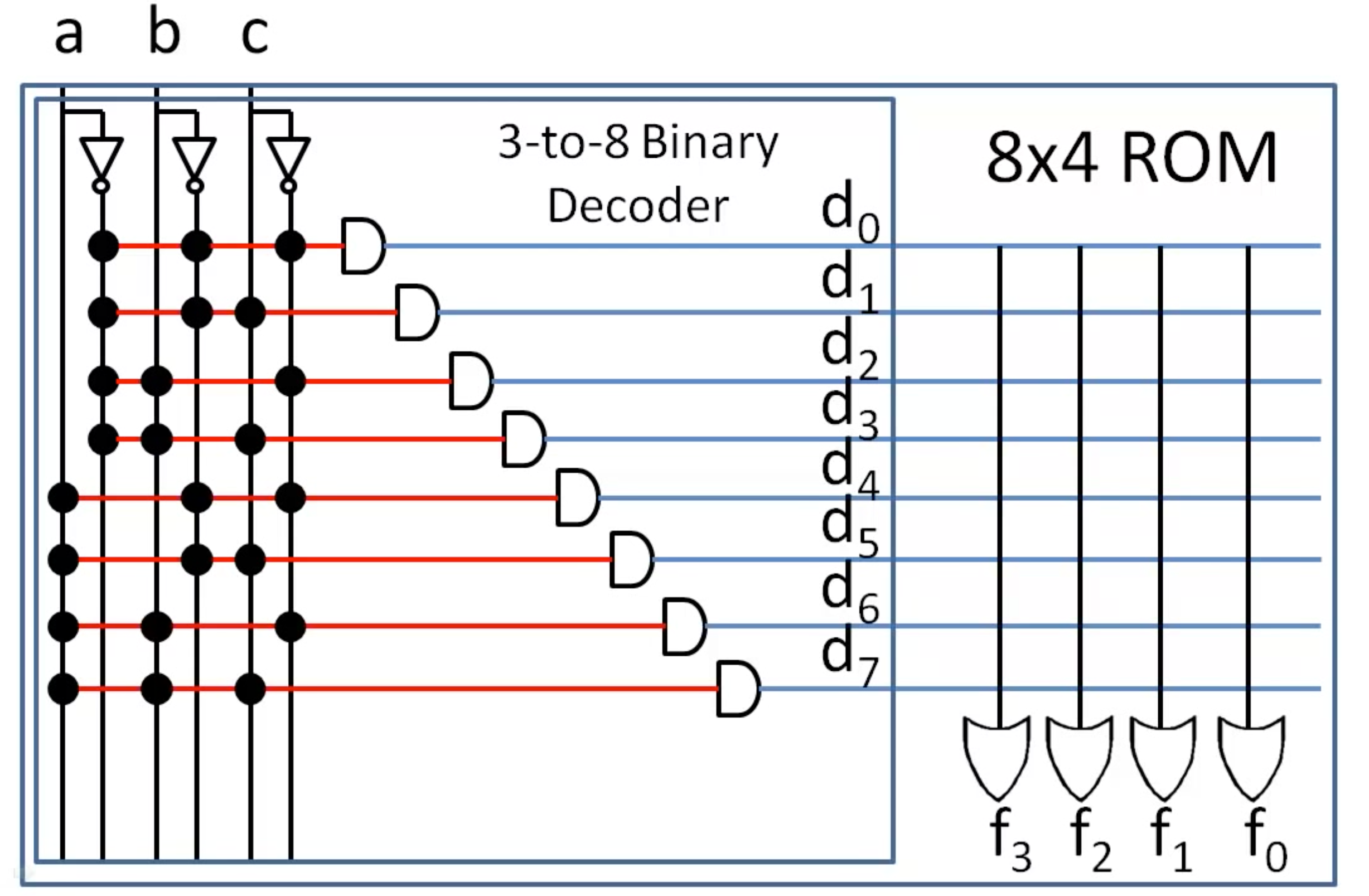Latest Posts

##### Effective Debugging Techniques in VHDL: Mastering the Art of Troubleshooting

Hot items

• 0.00

###### Resume Templates Mega Bundle For Electronics Engineers